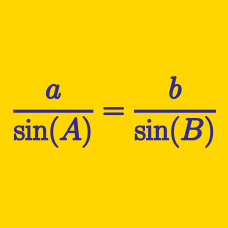Geometry

# Solving Triangles - Problem Solving

In triangle $$ABC$$, $$a=10$$, $$b=11$$ and $$c=12$$. If $$\cos A:\cos C$$ can be expressed as an irreducible ratio $$m:n$$, where $$m$$ and $$n$$ are positive integers, what is the value of $$m+n$$?

Let $$ABC$$ be a triangle and let $$a$$, $$b$$ and $$c$$ be the lengths of the sides opposite to the vertices $$A$$, $$B$$ and $$C$$, respectively. If $$a - 3b + c = 0$$ and $$2a + b - 2c = 0$$, the ratio $$\sin A : \sin B : \sin C$$ can be expressed as $$p:q:r$$, where $$p$$, $$q$$ and $$r$$ are coprime positive integers. What is the value of $$p+q+r$$?

In a convex quadrilateral $$ABCD,$$ we are given the following three angles and side length: $\angle B=\angle D=90^\circ, \angle A=45^\circ, \lvert\overline{AC}\rvert= 3\sqrt{2}.$ What is the measure of the diagonal $$\overline{BD} ?$$

Alice and Bob start walking at the same time from the same spot towards their respective directions, forming a $$60^\circ$$ angle. Their speeds are $$2$$m and $$3$$m per second, respectively. If the distance between them $$4$$ seconds after the start can be expressed as $$a\sqrt{b}$$, where $$b$$ is prime number, what is $$a+b$$?

In triangle $$ABC$$, $$\angle B=30^{\circ}$$, $$\angle C=90^{\circ}$$, and $$D$$ is a point on side $$BC$$ such that $$\overline{BD}=60$$ and $$\angle ADC=45^{\circ}$$. The length of side $$AC$$ can be expressed as $$p(1+\sqrt{3})$$. What is the value of $$p$$?

×